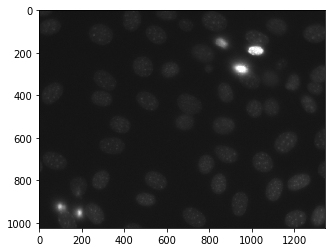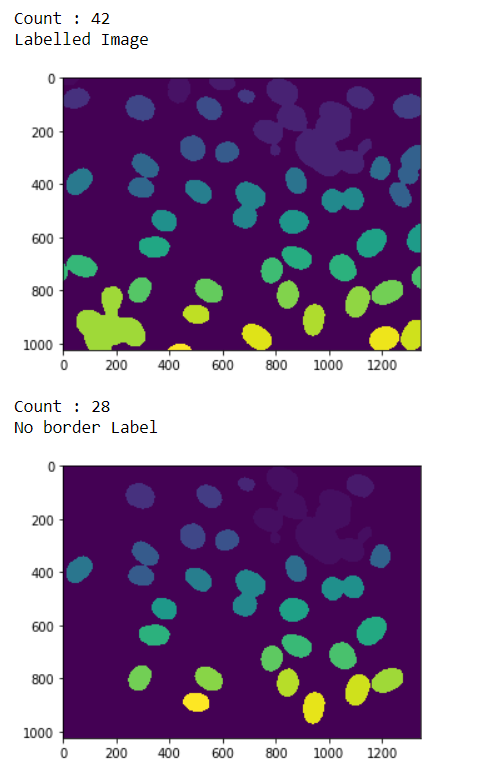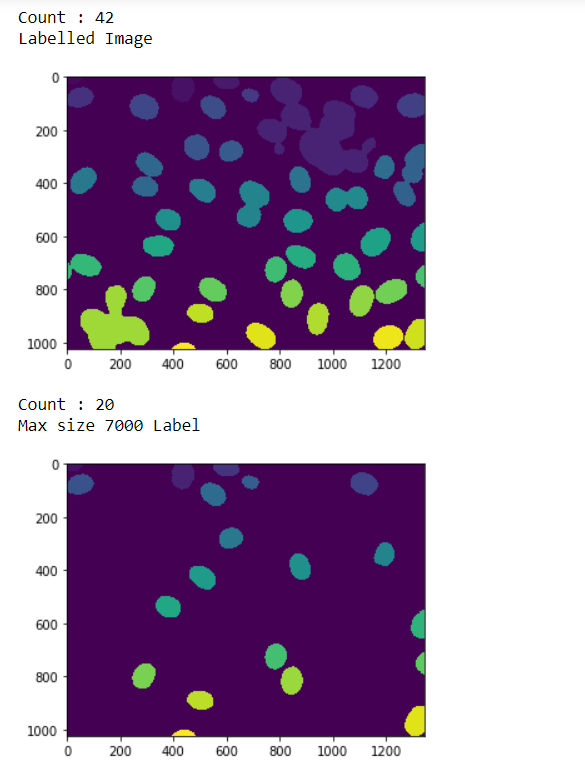# Mahotas – Filtering Labels

In this article we will see how we can filter the labels of the labelled image in mahotas. Filtering label is similar to implementing the relabeling feature but the difference is in filtering we will remove i.e filter the labels at the time of calling the filtering method and filtering will give us new labelled image and number of labels. We use mahotas.label method to label the image
For this we are going to use the fluorescent microscopy image from a nuclear segmentation benchmark. We can get the image with the help of command given below

`mahotas.demos.nuclear_image()`

Below is the nuclear_imageLabelled images are integer images where the values correspond to different regions. I.e., region 1 is all of the pixels which have value 1, region two is the pixels with value 2, and so on
In order to do this we will use mahotas.label.filter_labelled method

Syntax : mahotas.label.filter_labelled(label_image, filter1, filter2)
Argument : It takes labelled image object and filters as argument
Return : It returns the labelled image and integer i.e number of labels

Note : Filters can be border label filter, maximum size anything.
Example 1:

## Python3

 `# importing required libraries` `import` `mahotas` `import` `numpy as np` `from` `pylab ``import` `imshow, show` `import` `os`   `# loading nuclear image` `f ``=` `mahotas.demos.load(``'nuclear'``)`   `# setting filter to the image` `f ``=` `f[:, :, ``0``]`   `# setting gaussian filter` `f ``=` `mahotas.gaussian_filter(f, ``4``)`   `# setting threshold value` `f ``=` `(f> f.mean())`   `# creating a labelled image` `labelled, n_nucleus ``=` `mahotas.label(f)`   `# printing number of labels` `print``(``"Count : "` `+` `str``(n_nucleus))`   `# showing the labelled image` `print``(``"Labelled Image"``)` `imshow(labelled)` `show()`     `# filtering the label image` `# adding border filter` `relabelled, n_left ``=` `mahotas.labelled.filter_labelled(labelled, remove_bordering ``=` `True``)`   `# showing number of labels` `print``(``"Count : "` `+` `str``(n_left))`   `# showing the image` `print``(``"No border Label"``)` `imshow(relabelled)` `show()`

## Python3

 `# importing required libraries` `import` `mahotas` `import` `numpy as np` `from` `pylab ``import` `imshow, show` `import` `os`   `# loading nuclear image` `f ``=` `mahotas.demos.load(``'nuclear'``)`   `# setting filter to the image` `f ``=` `f[:, :, ``0``]`   `# setting gaussian filter` `f ``=` `mahotas.gaussian_filter(f, ``4``)`   `# setting threshold value` `f ``=` `(f> f.mean())`   `# creating a labelled image` `labelled, n_nucleus ``=` `mahotas.label(f)`   `# printing number of labels` `print``(``"Count : "` `+` `str``(n_nucleus))`   `# showing the labelled image` `print``(``"Labelled Image"``)` `imshow(labelled)` `show()`     `# filtering the label image` `# adding border filter` `relabelled, n_left ``=` `mahotas.labelled.filter_labelled(labelled, remove_bordering ``=` `True``)`   `# showing number of labels` `print``(``"Count : "` `+` `str``(n_left))`   `# showing the image` `print``(``"No border Label"``)` `imshow(relabelled)` `show()`

## Python3

 `# importing required libraries` `import` `mahotas` `import` `numpy as np` `from` `pylab ``import` `imshow, show` `import` `os`   `# loading nuclear image` `f ``=` `mahotas.demos.load(``'nuclear'``)`   `# setting filter to the image` `f ``=` `f[:, :, ``0``]`   `# setting gaussian filter` `f ``=` `mahotas.gaussian_filter(f, ``4``)`   `# setting threshold value` `f ``=` `(f> f.mean())`   `# creating a labelled image` `labelled, n_nucleus ``=` `mahotas.label(f)`   `# printing number of labels` `print``(``"Count : "` `+` `str``(n_nucleus))`   `# showing the labelled image` `print``(``"Labelled Image"``)` `imshow(labelled)` `show()`     `# filtering the label image` `# adding border filter` `relabelled, n_left ``=` `mahotas.labelled.filter_labelled(labelled, remove_bordering ``=` `True``)`   `# showing number of labels` `print``(``"Count : "` `+` `str``(n_left))`   `# showing the image` `print``(``"No border Label"``)` `imshow(relabelled)` `show()`

Output :Example 2:

## Python3

 `# importing required libraries` `import` `mahotas` `import` `numpy as np` `from` `pylab ``import` `imshow, show` `import` `os`   `# loading nuclear image` `f ``=` `mahotas.demos.load(``'nuclear'``)`   `# setting filter to the image` `f ``=` `f[:, :, ``0``]`   `# setting gaussian filter` `f ``=` `mahotas.gaussian_filter(f, ``4``)`   `# setting threshold value` `f ``=` `(f> f.mean())`   `# creating a labelled image` `labelled, n_nucleus ``=` `mahotas.label(f)`   `# printing number of labels` `print``(``"Count : "` `+` `str``(n_nucleus))`   `# showing the labelled image` `print``(``"Labelled Image"``)` `imshow(labelled)` `show()`     `# filtering the label image` `# adding max size filter` `relabelled, n_left ``=` `mahotas.labelled.filter_labelled(labelled, max_size ``=` `7000``)`   `# showing number of labels` `print``(``"Count : "` `+` `str``(n_left))`   `# showing the image` `print``(``"Max size 7000 Label"``)` `imshow(relabelled)` `show()`

## Python3

 `# importing required libraries` `import` `mahotas` `import` `numpy as np` `from` `pylab ``import` `imshow, show` `import` `os`   `# loading nuclear image` `f ``=` `mahotas.demos.load(``'nuclear'``)`   `# setting filter to the image` `f ``=` `f[:, :, ``0``]`   `# setting gaussian filter` `f ``=` `mahotas.gaussian_filter(f, ``4``)`   `# setting threshold value` `f ``=` `(f> f.mean())`   `# creating a labelled image` `labelled, n_nucleus ``=` `mahotas.label(f)`   `# printing number of labels` `print``(``"Count : "` `+` `str``(n_nucleus))`   `# showing the labelled image` `print``(``"Labelled Image"``)` `imshow(labelled)` `show()`     `# filtering the label image` `# adding max size filter` `relabelled, n_left ``=` `mahotas.labelled.filter_labelled(labelled, max_size ``=` `7000``)`   `# showing number of labels` `print``(``"Count : "` `+` `str``(n_left))`   `# showing the image` `print``(``"Max size 7000 Label"``)` `imshow(relabelled)` `show()`

Output :Whether you're preparing for your first job interview or aiming to upskill in this ever-evolving tech landscape, GeeksforGeeks Courses are your key to success. We provide top-quality content at affordable prices, all geared towards accelerating your growth in a time-bound manner. Join the millions we've already empowered, and we're here to do the same for you. Don't miss out - check it out now!

Previous
Next